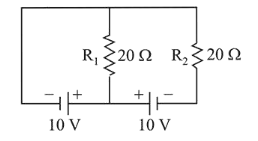Question:

In the given circuit the cells have zero internal resistance. The currents (in Amperes) passing through resistance $R_{1}$ and $R_{2}$ respectively, are:1. (1) 1,2

2. (2) 2,2

3. (3) $0.5,0$

4. (4) 0,1

Correct Option: , 3

Solution:

(3) Current passing through resistance $\mathrm{R}_{1}$,

$\mathrm{i}_{1}=\frac{\mathrm{v}}{\mathrm{R}_{1}}=\frac{10}{20}=0.5 \mathrm{~A}$ and, $\mathrm{i}_{2}=0$